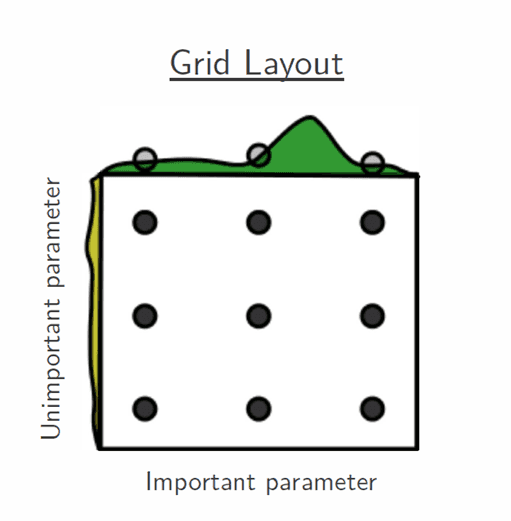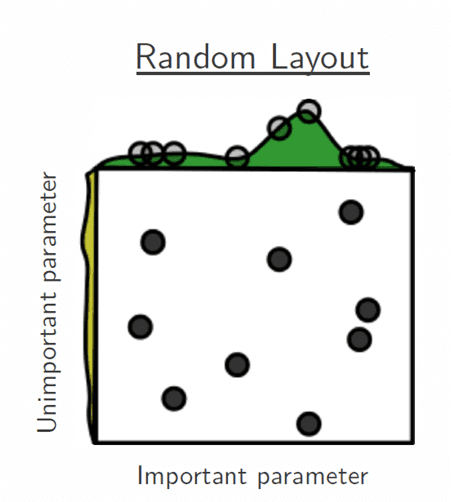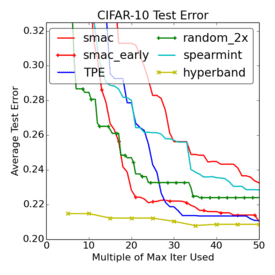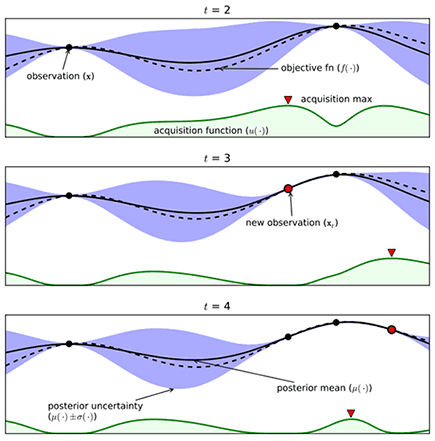Hyperparameter optimization is crucial for the success of a machine learning model or a deep learning architecture, since they heavily influence the behavior of the model learning. Often the search space of hyperparameters is fairly large for most machine learning and deep learning algorithms, that manually tuning is impossible.

The hyperparamters optimization literature is very rich in terms of algorithms, and many open source implementations are already existing. But finding good hyperparameters, does not only involve an efficient search algorithm over the space of possible hyperparameters, but also requires organization in order to review, compare, restart, or resume some of the tried experiments.

Polyaxon has a concept for suggesting hyperparameters and managing their results very similar to Google Vizier called experiment groups. An experiment group in Polyaxon defines a search algorithm, a search space, and a model to train.

### Search space

In order to perform hyperparameters tuning with Polyaxon the user must define a search space based on the supported methods in the matrix subsection:

— Discrete values

• values: a list of values, e.g.`['value1', 'value2', 'value3', 'value4']`
• range: [start, stop, step] same way you would define a range in python, e.g.`[1, 10, 2]`
• linspace: [start, stop, num] steps from start to stop spaced evenly on a linear scale, e.g.`[1, 10, 5]`
• logspace: [start, stop, num] steps from start to stop spaced evenly on a log scale, e.g. `[1, 10, 5]`
• geomspace: [start, stop, num] steps from start to stop, numbers spaced evenly on a a geometric progression, e.g. `[1, 10, 5]`

— Distributions

• pvalues: Draws a value_i from values with probability prob_i, e.g `[(value1, prob1), (value2, prob12), (value3, prob3), …]`
• uniform: Draws samples from a uniform distribution over the half-open interval `[low, high)`, e.g. [0, 1]
• quniform: Draws samples from a quantized uniform distribution over [low, high], `round(uniform(low, high) / q) * q`
• loguniform: Draws samples from a log uniform distribution over [low, high]
• qloguniform: Draws samples from a quantized log uniform distribution over [low, high]
• normal: Draws random samples from a normal (Gaussian) distribution defined by [loc, scale]
• qnormal: Draws random samples from a quantized normal (Gaussian) distribution defined by [loc, scale]
• lognormal: Draws random samples from a log normal (Gaussian) distribution defined by [loc, scale]
• qlognormal: Draws random samples from a quantized log normal (Gaussian) distribution defined by [loc, scale]

Example:

``````matrix:
lr:
logspace: 0.01:0.1:5

loss:
values: [MeanSquaredError, AbsoluteDifference]``````

### Search algorithms

Polyaxon supports a couple of algorithms for traversing the search space, Grid search, Random Search, Hyperband, and Bayesian Optimization.The default search algorithm used by Polyaxon when the user does not define a search algorithm. It is essentially an exhaustive search through a manually specified set of hyperparameters. The user can possibly limit the number of experiments created by providing `n_experiments`

For example, if the search space contains 20 unique hyperparameters combination, and the user specify `n_experiments` the search algorithm will only visit the first 10 combinations. Here’s an example to illustrate that:

``````settings:

grid_search:
n_experiments: 10

concurrency: 2

matrix:
learning_rate:
linspace: 0.001:0.1:5
dropout:
values: [0.25, 0.3]
activation:
values: [relu, sigmoid]``````Random search creates a number of unique experiments by sampling randomly from a search space. Random search is a competitive method for black-box parameter tuning in machine learning.

Here’s a concrete example where the random search algorithm will try 30 unique experiments based on the space search defined in the matrix subsection.

``````settings:
random_search:
n_experiments: 30
concurrency: 2

matrix:
learning_rate:
uniform: 0.001:0.01
dropout:
values: [0.25, 0.3, 0.5]
activation:
pvalues: [(relu, 0.2), (sigmoid, 0.8)]``````

#### HyperbandHyperband: A Novel Bandit-Based Approach to Hyperparameter Optimization

Hyperband is a relatively new method for tuning iterative algorithms. It performs random sampling and attempts to gain an edge by using time spent optimizing in the best way. The algorithm tries a large number of random configurations/experiments, then decides which configurations to keep based on their progress.

In order to configure this search algorithm correctly, you need to have as one of the hyperparameters, a resource, this could be the number of steps or epochs, and a metric that you want to maximize or minimize. You can also indicate if the experiments should be restarted from scratch or resumed from the last check point.

The way Hyperband works is by discarding poor performing configurations leaving more resources for more promising configurations during the successive halving.

In order to use Hyperband correctly, you must define a hyperparameter called `resource` that the algorithm will increase iteratively. Here’s an example of resource definitions:

``````resource:
name: num_steps
type: int``````

You can also have a resource with type float.

Another important concept is the metric to optimize, for example:

``````metric:
name: loss
optimization: minimize``````

or

``````metric:
name: accuracy
optimization: maximize``````

Here’s a concrete example:

``````settings:
hyperband:
max_iter: 81
eta: 3
resource:
name: num_steps
type: int
metric:
name: loss
optimization: minimize
resume: False  concurrency: 2

matrix:
learning_rate:
uniform: 0.001:0.01
dropout:
values: [0.25, 0.3, 0.5]
activation:
pvalues: [(relu, 0.2), (sigmoid, 0.8)]``````

#### Bayesian OptimizationGaussian process approximation of objective function

Bayesian optimization is an extremely powerful technique. The main idea behind it is to compute a posterior distribution over the objective function based on the data, and then select good points to try with respect to this distribution.

The way Polyaxon performs bayesian optimization is by measuring the expected increase in the maximum objective value seen over all experiments in the group, given the next point we pick.

Since the bayesian optimization leverages previous experiments, the algorithm requires a metric to optimize (`maximize` or `minimize`).

To use bayesian optimization the user must define a utility function.

A couple of acquisition function are currently supported:

• `ucb`: Upper Confidence Bound,
• `ei`: Expected Improvement
• `poi`: Probability of Improvement

When using `ucb` as acquisition function, a tunable parameter `kappa` is also required, to balance exploitation against exploration, increasing kappa will make the optimized hyperparameters pursuing exploration.

When using `ei` or `poi` as acquisition function, a tunable parameter `eps` is also required, to balance exploitation against exploration, increasing epsilon will make the optimized hyperparameters more spread out across the whole range.

In order to use Bayesian Optimization, the user can only choose matrix options that provide discrete values, e.g. range, linspace, categorical values …, or the uniform distribution.

Here’s a concrete example:

``````settings:

bo:
n_iterations: 5
n_initial_trials: 10
metric:
name: loss
optimization: minimize
utility_function:
acquisition_function: ucb
kappa: 2.576
gaussian_process:
kernel: matern
length_scale: 1.0
nu: 1.9
n_restarts_optimizer: 0  concurrency: 2

matrix:
learning_rate:
uniform: [0.001, 0.01]
dropout:
values: [0.25, 0.3]
activation:
values: [relu, sigmoid]``````

### Early stopping

Sometimes it does not make sense to continue searching the hyperparameters space, if one of the experiments’ metrics have reached a certain threshold. This why Polyaxon provides also an early stopping mechanism.

Early stopping defines a list of metrics and the values for these metrics to stop the search algorithm when they are reached.

Example:

``````early_stopping:
- metric: accuracy
value: 0.9
optimization: maximize
- metric: loss
value: 0.05
optimization: minimize``````

### Examples

You can find real some configuration examples of these algorithms in our quick-start github repo.

### Conclusion

Hopefully this blogpost gave you a little insight on how Polyaxon abstracts state-of-the-art algorithms for hyperparameters tuning so you can automate and develop powerful machine learning models with far less effort.

Polyaxon will continue to implement more algorithms and provide simple interfaces. And as always, let us know if you have any feedback on this and other features on Polyaxon.